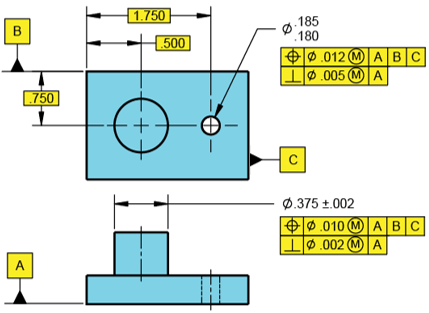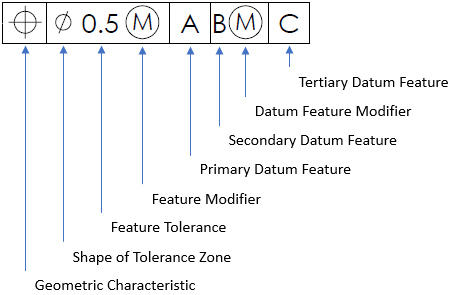## MMC Hole’s Virtual Size

Answer the following questions based on the figure shown.
1. What is the hole’s MMC size?
2. What is the hole’s Virtual Size relative to Datums ABC?
3. What is the hole’s Virtual Size relative to Datum A?Hint
Breaking down the parts of a feature control frame:Hint 2
The virtual condition is used to analyze the clearance distance between mating parts. It is a constant boundary generated by a combination of a feature of size’s specified MMC or LMC and the geometric tolerance for that material condition.
Breaking down the parts of a feature control frame:The problem statement specifies a Max Material Condition (MMC) feature modifier. A MMC modifier is the condition in which a feature of size contains the maximum amount of material within the stated limits of size. For a hole, MMC is the dimension that produces the smallest cutout. Thus,
$$MMC_{hole}=.180$$$The virtual condition is used to analyze the clearance distance between mating parts. It is a constant boundary generated by a combination of a feature of size’s specified MMC or LMC and the geometric tolerance for that material condition. In summary, For an Internal Feature (Hole) with Geo Tol at MMC: • Inner Boundary (IB) = MMC (think smallest hole) - geo tol = Virtual Size/Condition For an External Feature (Pin) with Geo Tol at MMC: • Outer Boundary (OB) = MMC (think largest pin) + geo tol = Virtual Size/Condition where $$geo\:tol$$ is the stated value in the feature control frame. In the feature control frame, the positional tolerance is the one associated with Datums ABC. Thus, the hole’s virtual size is: $$.180-.012=.168$$$
In the feature control frame, the perpendicularity tolerance is the one associated with Datum A only. Thus, the hole’s virtual size is:
$$.180-.005=.175$$\$
1. .180
2. .168
3. .175